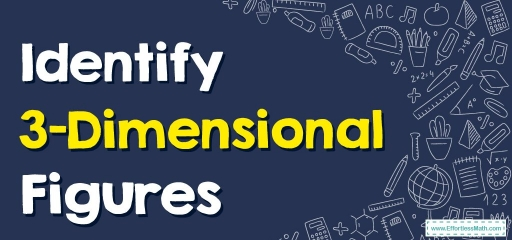# Identify 3–Dimensional FiguresA $$3D$$ shape is a solid shape or object with $$3$$ dimensions (length, width, and height), instead of $$2$$-dimensional objects having just length and width.

Additional significant terms linked to $$3D$$ geometric shapes could be faces, vertices, and edges. These have got depth, therefore they inhabit some volume.

A few $$3D$$ shapes have $$2D$$ shapes for their bases or cross-sections. For instance, cubes have all their faces shaped like squares.

A $$3D$$ shape is categorized into numerous classifications. A few have a curved surface; a few are shaped like a pyramid or a prism.

A few cases of $$3D$$ shapes are cubes, cuboids, cones, and cylinders.

## Spheres

Spheres are shaped like a circle. They are $$3D$$ geometric shapes with all their points on their surfaces which are equidistant from their centers.

## Cubes and Cuboids

Cubes and cuboids are $$3$$-dimensional shapes ($$3D$$ shapes) with an identical amount of faces, edges, and vertices. The key difference in-between cubes and cuboids is that for cubes, all their 6 faces are squares, while for cuboids, all their 6 faces are rectangles.

Cubes and cuboids dwell in various volumes and have various surface areas. A cube’s length, width, and height are identical, whilst, for cuboids, their lengths, heights, and widths vary.

## Cylinders

Cylinders are $$3D$$ shapes with $$2$$ circular faces, one is at its top and one is at its bottom, and there’s $$1$$ curved surface. Cylinders have a height as well as a radius. A cylinder’s height is the perpendicular distance in-between its top and bottom faces.

## Cones

Cones are yet another $$3$$-dimensional shape ($$3D$$ shape) with a flat base (that is round in shape) as well as a pointed tip at its top. The pointed end of its top is termed an ‘Apex’.

The cone additionally has a curved surface. Comparable to cylinders, cones may additionally get classed as a right circular cone as well as an oblique cone.

## Pyramids

Pyramids are polyhedrons having a polygon base as well as an apex having flat faces and straight edges. Dependent on their apex alignment in conjunction with the base’s center, they may be classified as oblique and regular pyramids.

## Prisms

Prisms are solids having the same polygon ends as well as they have flat parallelogram sides.

### Identify 3–Dimensional Figures – Example 1:

Write the name of the shape.

Solution:

This shape has a flat base as well as a pointed tip at its top. So, it’s a cone

### Identify 3–Dimensional Figures – Example 2:

Write the name of the shape.

Solution:

This shape has the same polygon ends as well as flat parallelogram sides. So, it’s a prism. Also, this is a prism in the shape of a triangle so it’s a triangular prism.

## Exercises for Identify 3–Dimensional Figures

Write the name of each shape.

1)

2)

1. $$\color{blue}{Cube}$$
2. $$\color{blue}{Cylinder}$$

### What people say about "Identify 3–Dimensional Figures - Effortless Math: We Help Students Learn to LOVE Mathematics"?

No one replied yet.

X
30% OFF

Limited time only!

Save Over 30%

SAVE $5 It was$16.99 now it is \$11.99